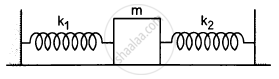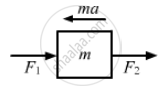# Both the Springs Shown in Figure (5−E2) Are Unstretched. If the Block is Displaced by a Distance X And Released, What Will Be the Initial Acceleration? - Physics

Sum

Both the springs shown in the following figure are unstretched. If the block is displaced by a distance x and released, what will be the initial acceleration?#### Solution

Let the block m be displaced towards left by displacement x.$\therefore F_1 = -K_1X$  (compressed)
$F_2 = -K_2X$  (expanded)
$ma = F_1 + F_2$
$\Rightarrow a = \frac{- x\left( k_1 + k_2 \right)}{m}$
i.e.  $\left( k_1 + k_2 \right)\frac{x}{m}$ opposite the displacement or towards the mean position.

Concept: Newton’s Second Law of Motion
Is there an error in this question or solution?

#### APPEARS IN

HC Verma Class 11, Class 12 Concepts of Physics Vol. 1
Chapter 5 Newton's Laws of Motion
Q 10 | Page 79